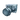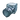## FANDOM

634 Pages

The Thruster is a type of block in Space Engineers.

The Primary function of a thruster is to provide ships with the ability to move. When turned on, either by use of the movement keys in a cockpit, Remote Control, or using the thruster's manual override in the control panel, the thruster applies force in the direction opposite to it's exhaust. Thrusters can only push and pull ships in their respective directions, so it's recommended to have thrusters in all 6 directions for conventional ship designs.

The thruster is also used to counteract other forces via the use of inertial dampeners, when enabled, thrusters will be applied as neccesary to slow ships down. They will also fight against planetary gravity, so ensure that you have enough skyward thrusters on atmospheric ships to achieve this.

While they can be placed anywhere on a ship, thrusters require 4-5 blocks of clear space to avoid damaging other objects. Small ship thrusters, however, should not damage heavy armour blocks. Thruster Damage can be toggled on and off in world settings, allowing for different ship designs.

Note: Prior to Update 01.022, thrusters did not require open space to function.

## Capacity

When active, inertial dampeners can use 100% of the maximum capacity of all necessary thrusters. However, when fired via movement keys, thrusters will only operate at 2/3 maximum capacity. The listed power and force ratings on theLarge Thruster andSmall Thruster pages reflect maximum capacity. When attempting calculations for a manual burn, remember to multiply these values by 2/3.

## Calculating acceleration

According to physics, we know that force = mass x acceleration. Using algebra, we can then determine that acceleration = force / mass. But what does this mean, exactly?

#### Units

• Force: Newtons (N), 1 N = 1 kgm/s2
• Mass: Kilograms (kg)
• Acceleration: meters per second per second (m/s2)

#### Equation

To calculate the acceleration of your ship, use the following formula:

 $a= \frac{(N_l F_l + N_s F_s)}{m}$ Where: $a$ : Acceleration (m/s2) $N_l$ : Number of large thrusters $F_l$ : Force of each large thruster (N) $N_s$ : Number of small thrusters $F_s$ : Force of each small thruster (N) $m$ : Mass of the ship (kg)

### Online calculator

http://se-speed.ga online calculator for easy calculation.

### Example

Let's assume we have a 15000kg small ship with one large thruster and four small ones pointing aft. We want to know how fast we'll accerate when we press 'w'.

 Steps: 1. Calculate small thruster force$N_s F_s = 4 \times 12,110 \text{N} = 48,440 \text{N}$ 2. Add the large thruster.$N_s F_s + N_l \times F_l = 48,440 \text{N} + 1 \times 145,500 = 193,940 \text{N}$ 3. Calculate acceleration.$a=\frac{ 193,940 \text{N} }{ 15,000 \text{kg} } = 12.93 \text{m/s}^{2}$

## Calculating time to velocity

Sometimes, it may be important to know how long it will take to accelerate to a particular speed. To calculate the amount this, you first need the rate of acceleration calculated above. Then, since we know that velocity = acceleration * time, you can use algebra to find the following equation:

### Equation

 $t=\frac {\Delta v} {a}$ $\Delta v=v_f - v_i$ Where: $\Delta v$ : Change in velocity (m/s) $a$ : Acceleration (m/s2) $v_f$ : Final velocity (m/s) $v_i$ : Initial velocity (m/s)

### Example

Our ship in the previous example had an acceleration of 12.93 m/s2. If we want to know how long it takes to accelerate from a stop up to the game's speed limit, we simply fill in the equation:

 Steps: 1. Get delta-v.$v=105 \text{m/s} - 0\text{m/s}$ 2. Calculate time.$t=\frac {105 \text{m/s} } { 12.93 \text{m/s}^2 } = 8.1 \text{s}$

## Distance traveled

Another useful thing to know is how far you'll travel while accelerating. For example, if we plan on docking with a station or carrier, it's good to know exactly how close we can get before we have to start slowing down. For this, we need to know our initial velocity, our acceleration, and the time.

### Equation

 $d=v_it + \frac {at^2} {2}$ Where: $d$ : Distance (m) $v_i$ : Initial velocity (m/s) $a$ : Acceleration (m/s2) $t$ : Time (s)

### Example

Let's say we've got a small ship up near the speed limit at 100m/s. We want to slow down to about 10m/s in order to dock safely. Our ship isn't really designed for rapid deceleration, so our forward thrusters only generate an acceleration of 15m/s2. (Note: since we're decelerating, we use -15m/s2.)

 Steps: 1. Calculate how much time it'll take.$t=\frac {10\text{m/s} - 100\text{m/s} } {-15\text{m/s}^2}$ 2. $t=\frac {-90\text{m/s} } {-15\text{m/s}^2}$ 3. $t=6\text{s}$ 4. Then figure out how much distance we need.$d=100\text{m/s}(6\text{s}) + \frac {-15\text{m/s}^2 \ 6^2\text{s} } {2}$ 5. $d=600\text{m} + \frac {-15\text{m/s}^2 \ 36\text{s}^2} {2}$ 6. $d=600\text{m} + \frac {-540\text{m} } {2}$ 7. $d=600\text{m} - 270\text{m}$ 8. $d=330\text{m}$

So we need at least 330m to drop down to our safe speed.

When doing these calculations, it's important to remember that you get more power from inertial dampeners than from manual thrusters. It's even more important to remember your ship can have a different acceleration depending on how many thrusters point away from where you're moving.

Since most ships have more aft thrusters than fore thrusters, you can run into problems if you base all your calculations off aft inertial dampeners. You may well find yourself merging your hull into the side of a station.

## Multiple thrust vectors

Community content is available under CC-BY-SA unless otherwise noted.